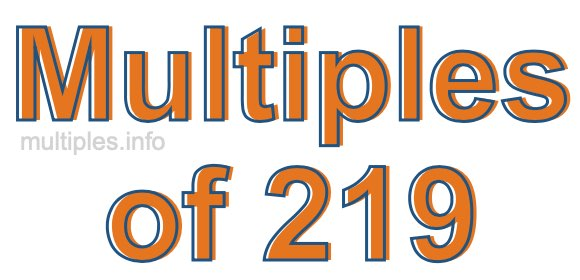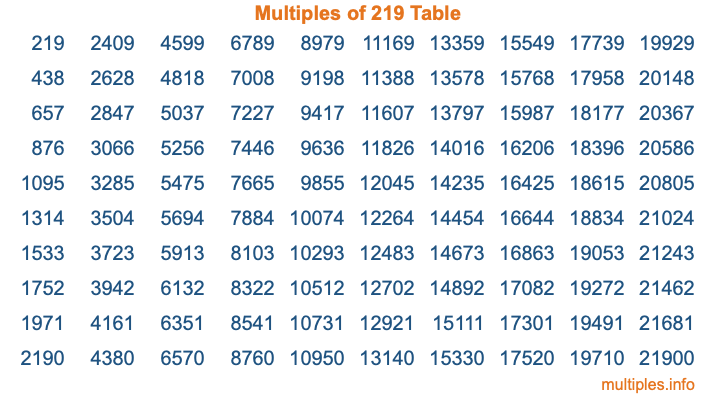Multiples of 219Welcome to the Multiples of 219 page. Here we will first teach you everything you will ever need to know about the multiples of 219, and then give you a study guide summary of everything we taught you to make sure you remember it all. Use this page to look up facts and learn information about the multiples of 219. This page will make you a multiples of two hundred nineteen expert!

Definition of Multiples of 219
Multiples of 219 are all the numbers that when divided by 219 equal an integer. Each of the multiples of 219 are called a multiple. A multiple of 219 is created by multiplying 219 by an integer.

Therefore, to create a list of multiples of 219, you start with 1 multiplied by 219, then 2 multiplied by 219, then 3 multiplied by 219, and so on for as long as you want. Thus, the list of the first five multiples of 219 is 219, 438, 657, 876, and 1095. To see a larger list of multiples of 219, see the printable image of Multiples of 219 further down on this page. We also have a category where you can choose any nth multiple of 219.

Multiples of 219 Checker
The Multiples of 219 Checker below checks to see if any number of your choice is a multiple of 219. In other words, it checks to see if there is any number (integer) that when multiplied by 219 will equal your number. To do that, we divide your number by 219. If the the quotient is an integer, then your number is a multiple of 219.

Is  a multiple of 219?

Least Common Multiple of 219 and ...
A Least Common Multiple (LCM) is the lowest multiple that two or more numbers have in common. This is also called the smallest common multiple or lowest common multiple and is useful to know when you are adding our subtracting fractions. Enter one or more numbers below (219 is already entered) to find the LCM.

Check out our LCM Calculator if you need more details about the Least Common Multiple or if you need the LCM for different numbers for adding and subtraction fractions.

nth Multiple of 219
As we stated above, 219 is the first multiple of 219, 438 is the second multiple of 219, 657 is the third multiple of 219, and so on. Enter a number below to find the nth multiple of 219.

th multiple of 219

Multiples of 219 vs Factors of 219
219 is a multiple of 219 and a factor of 219, but that is where the similarities end. All postive multiples of 219 are 219 or greater than 219. All positive factors of 219 are 219 or less than 219.

Below is the beginning list of multiples of 219 and the factors of 219 so you can compare:

Multiples of 219: 219, 438, 657, 876, 1095, etc.

Factors of 219: 1, 3, 73, 219

As you can see, the multiples of 219 are all the numbers that you can divide by 219 to get a whole number. The factors of 219, on the other hand, are all the whole numbers that you can multiply by another whole number to get 219.

It's also interesting to note that if a number (x) is a factor of 219, then 219 will also be a multiple of that number (x).

Multiples of 219 vs Divisors of 219
The divisors of 219 are all the integers that 219 can be divided by evenly. Below is a list of the divisors of 219.

Divisors of 219: 1, 3, 73, 219

The interesting thing to note here is that if you take any multiple of 219 and divide it by a divisor of 219, you will see that the quotient is an integer.

Multiples of 219 Table
Below is an image of the first 100 multiples of 219 in a table. The table is in chronological order, column by column. The first column has the first ten multiples of 219, the second column has the next ten multiples of 219, and so on.The Multiples of 219 Table is also referred to as the 219 Times Table or Times Table of 219. You are welcome to print out our table for your studies.

Negative Multiples of 219
Although not often discussed or needed in math, it is worth mentioning that you can make a list of negative multiples of 219 by multiplying 219 by -1, then by -2, then by -3, and so on, to get the following list of negative multiples of 219:

-219, -438, -657, -876, -1095, etc.

Multiples of 219 Summary
Below is a summary of important Multiples of 219 facts that we have discussed on this page. To retain the knowledge on this page, we recommend that you read through the summary and explain to yourself or a study partner why they hold true.

There are an infinite number of multiples of 219.

A multiple of 219 divided by 219 will equal a whole number.

219 divided by a factor of 219 equals a divisor of 219.

The nth multiple of 219 is n times 219.

The largest factor of 219 is equal to the first positive multiple of 219.

219 is a multiple of every factor of 219.

219 is a multiple of 219.

A multiple of 219 divided by a divisor of 219 equals an integer.

219 divided by a divisor of 219 equals a factor of 219.

Any integer times 219 will equal a multiple of 219.

Multiples of a Number
Here you can get the multiples of another number, all with the same attention to detail as we did for multiples of 219 on this page.

Multiples of
Multiples of 220
Did you find our page about multiples of two hundred nineteen educational? Do you want more knowledge? Check out the multiples of the next number on our list!